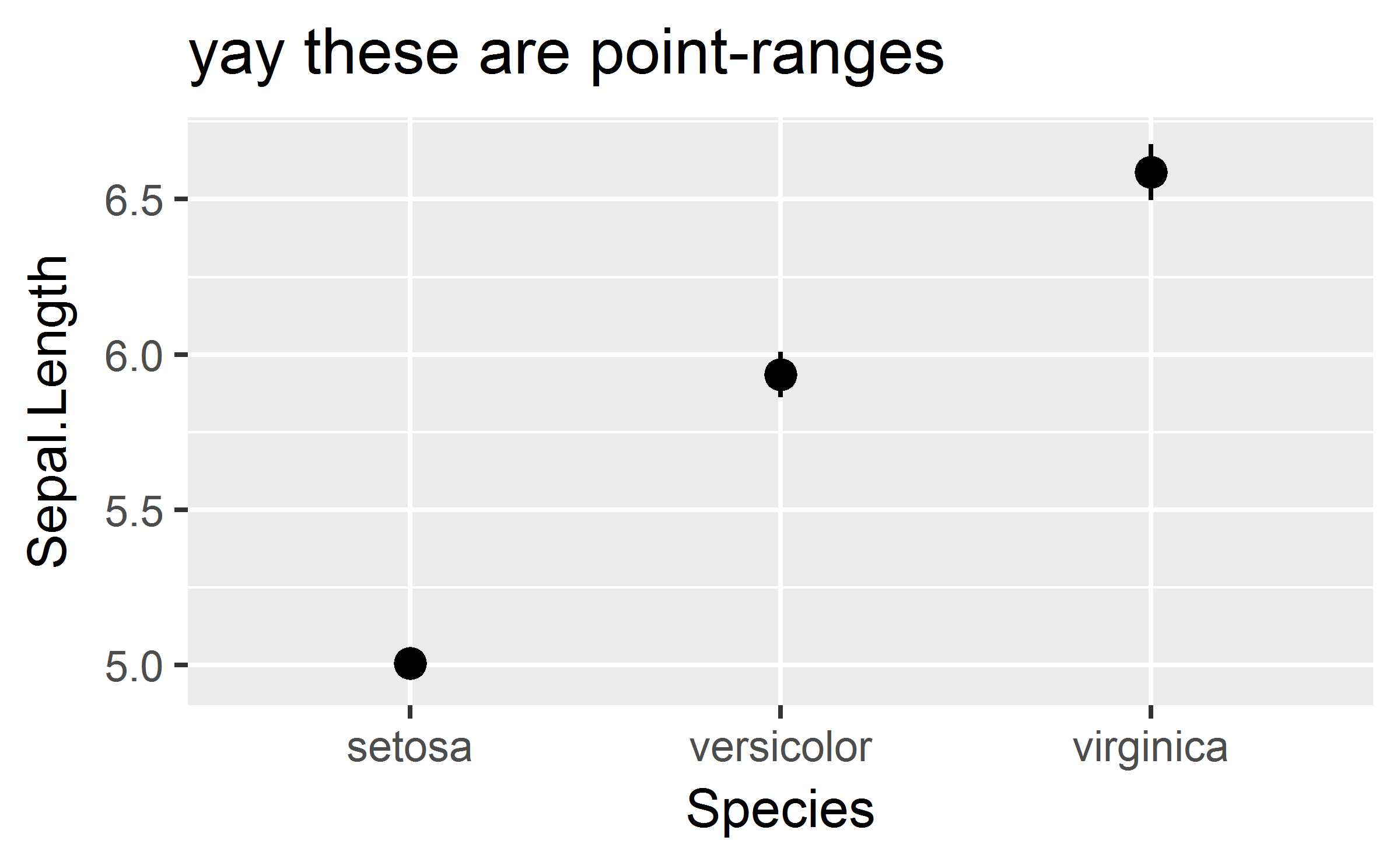I wrote a function today to wrap another function and call that function with some default arguments. Some of these defaults are “soft”: the user can overwrite them. Others are “hard”: the user cannot overwrite them.

library(ggplot2)

wrap_with_defaults <- function(func, hard_defaults, soft_defaults) {
function(...) {
dots <- list(...)
# overwrite soft defaults with user options
# then overwrite with hard defaults
args <- modifyList(modifyList(soft_defaults, dots), hard_defaults)
do.call(func, args)
}
}

The function uses a “closure”. We give wrap_with_defaults() some data (a target function and some default values), and it returns a new function which encloses that data. In this case, the new function remembers the target function and the default values we want to use with that target function.

This is the intended usage, and it works flawlessly.

stat_mean_se <- wrap_with_defaults(
stat_summary,
hard_defaults = list(fun.data = mean_se),
# pass in a variable as a default
soft_defaults = list(geom = "pointrange")
)

ggplot(iris) +
aes(x = Species, y = Sepal.Length) +
stat_mean_se() +
ggtitle("oooh look at these point-ranges")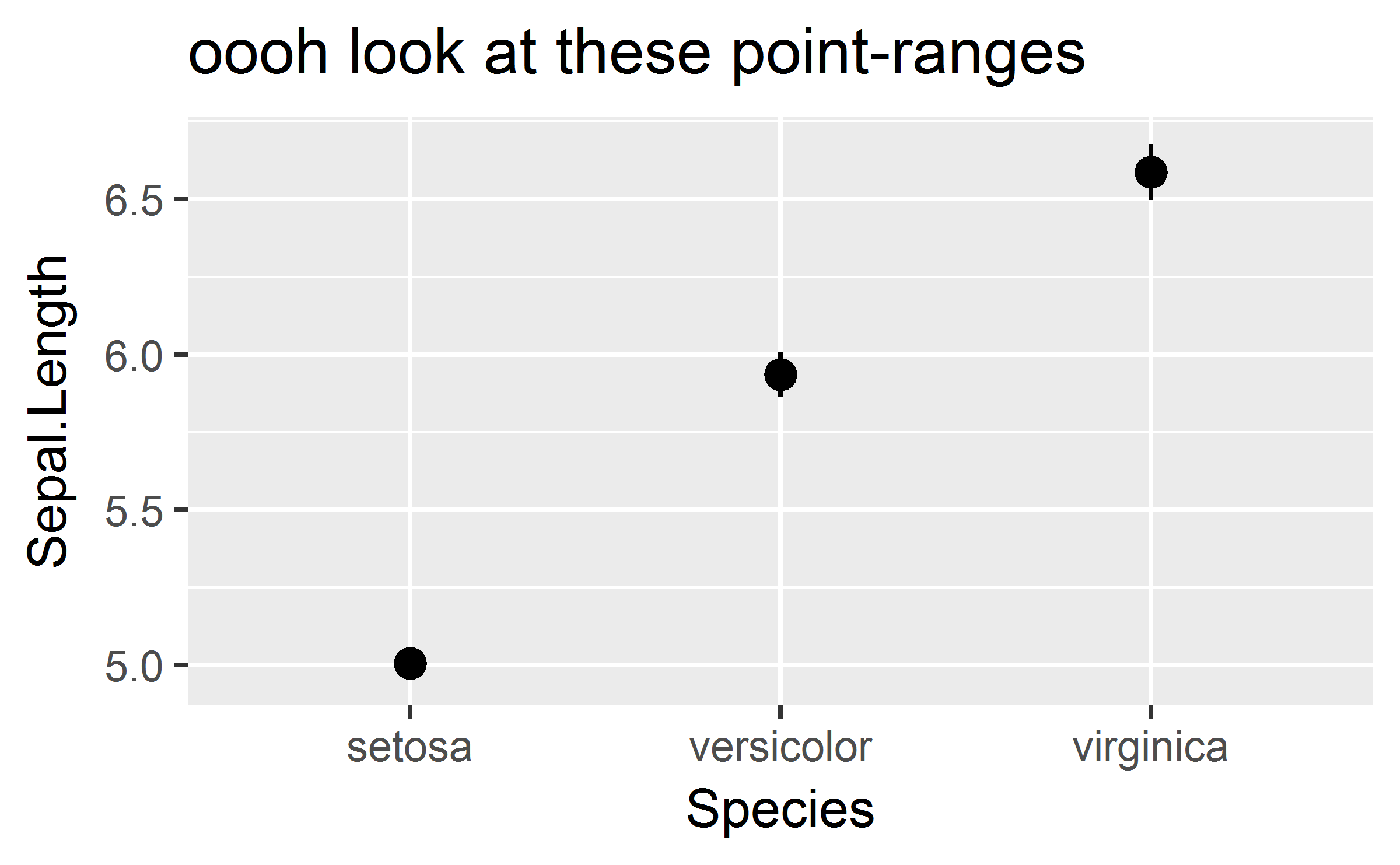But there’s a subtle bug in my function-wrapping function. Suppose we get one of the default values from a variable.

geom <- "pointrange"

stat_mean_se2 <- wrap_with_defaults(
stat_summary,
hard_defaults = list(fun.data = mean_se),
# pass in a variable as a default
soft_defaults = list(geom = geom)
)

And that variable changes.

geom <- "errorbar"

Then something funny happens.

ggplot(iris) +
aes(x = Species, y = Sepal.Length) +
stat_mean_se2() +
ggtitle("ahhh these aren't point-ranges")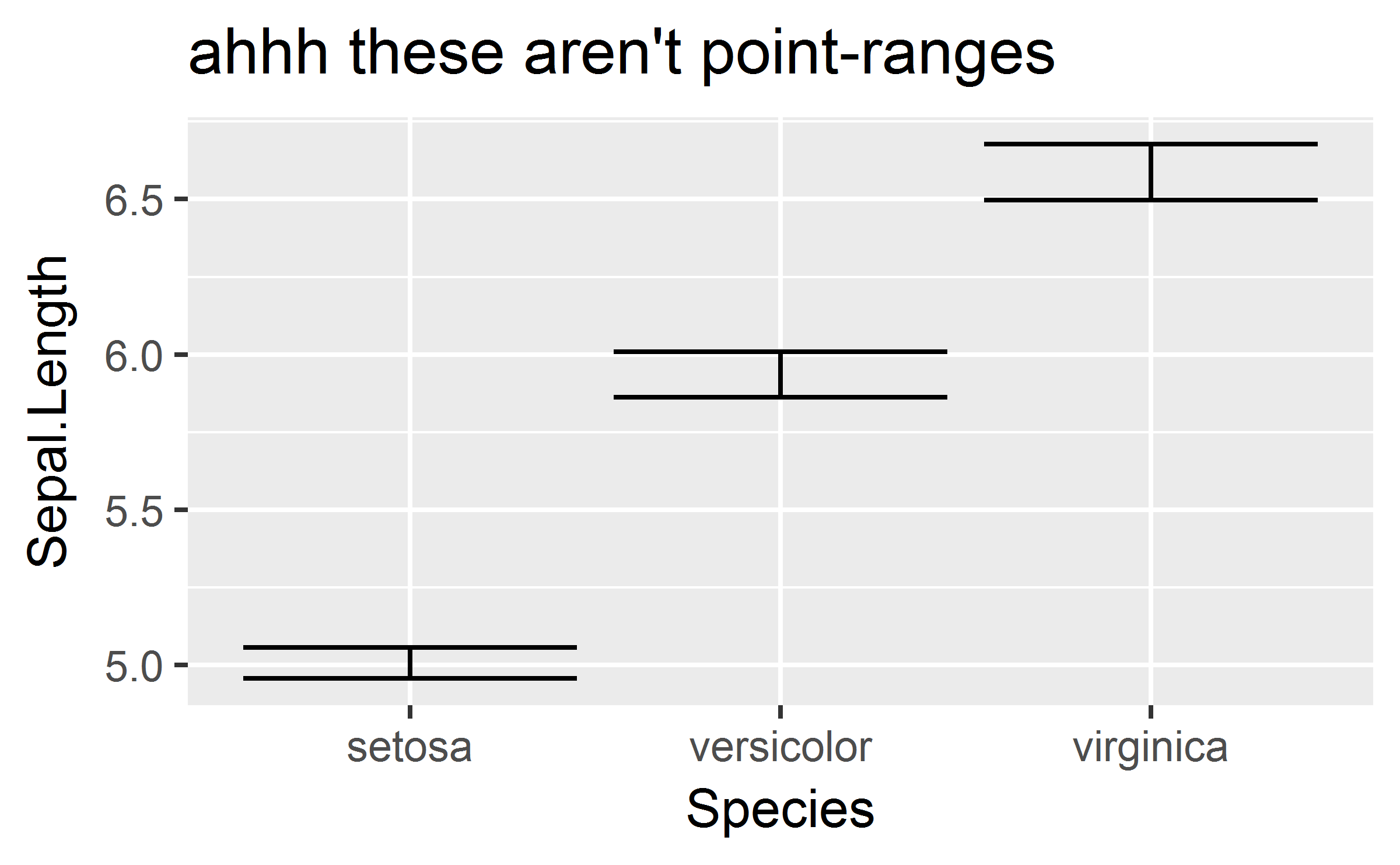The plot used the updated value of the geom variable! Maybe updating geom again will update it again?

geom <- "pointrange"

ggplot(iris) +
aes(x = Species, y = Sepal.Length) +
stat_mean_se2() +
ggtitle("ahhh these *still* aren't point-ranges")

geom <- "pointrange"
ggplot(iris) +
aes(x = Species, y = Sepal.Length) +
stat_mean_se2(color = "blue") +
ggtitle("please draw some point-ranges")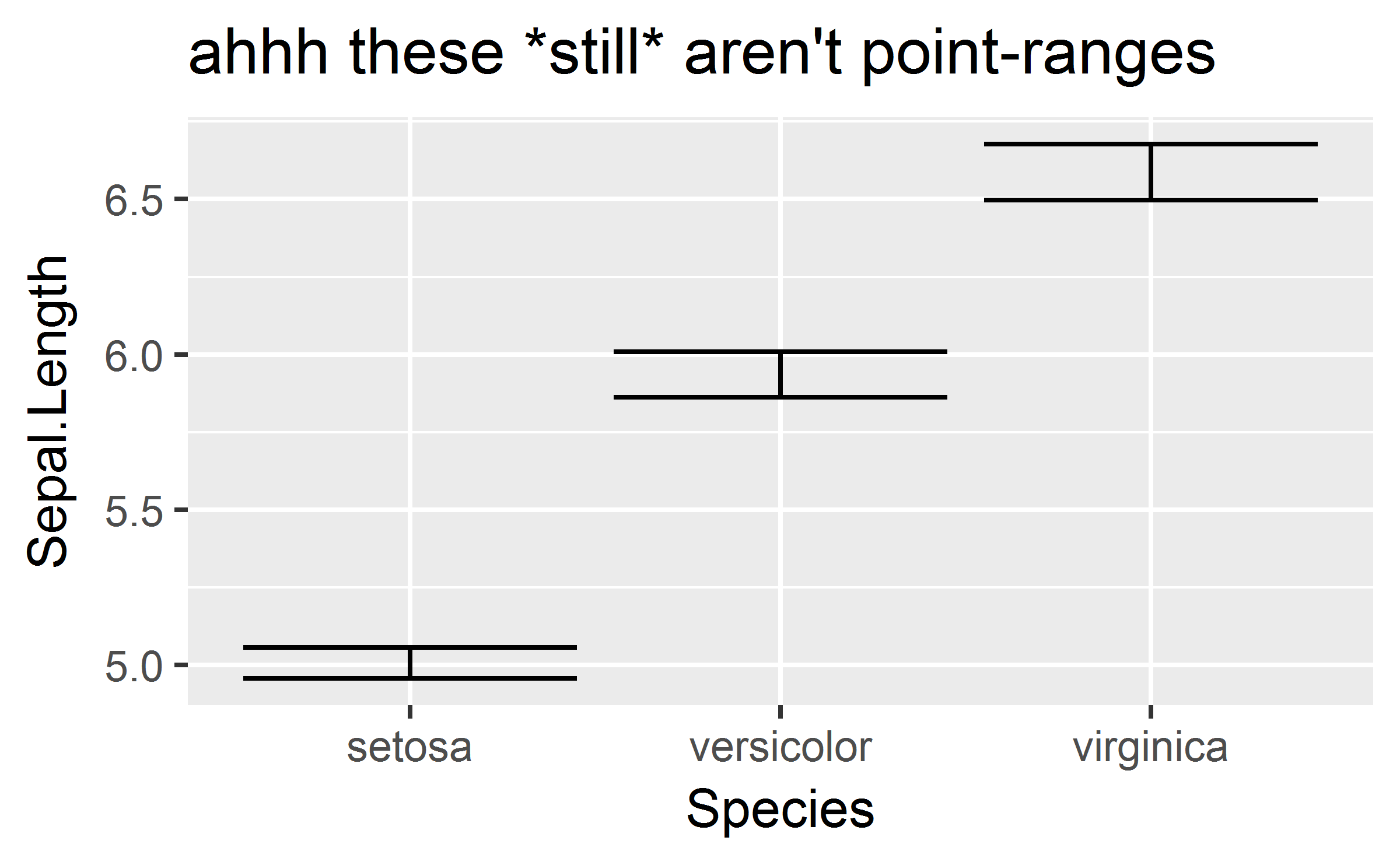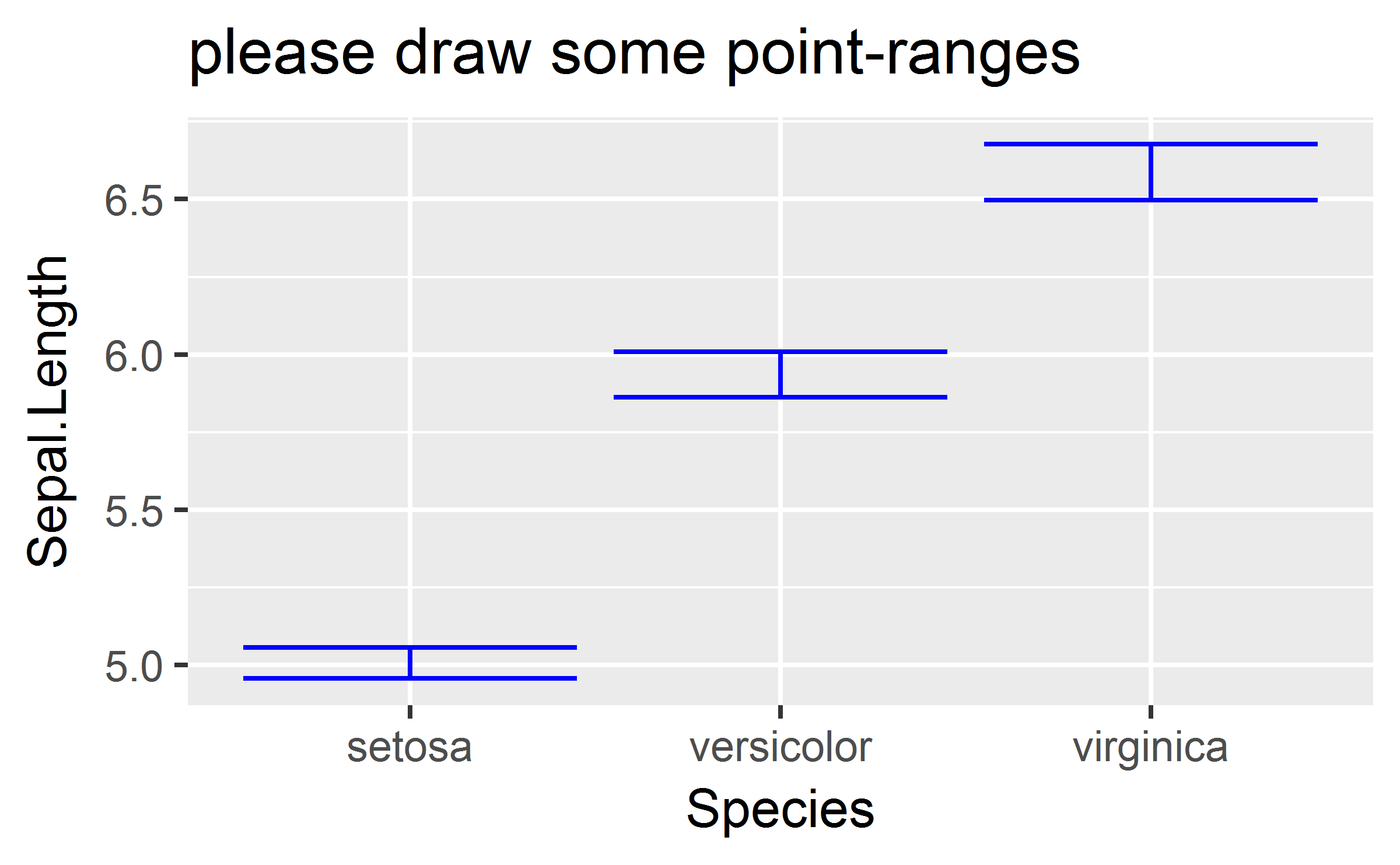Nope!

What happen is that default values were enclosed inside the newly created function, but they were lazily evaluated. The values of the defaults were only set when we finally used them, which was the first time the function was called. That’s why changing geom the first time worked. Once that value was evaluated, the value was fixed inside the closure so that once-evaluated value of geom would be used on all subsequent calls of the function.

The solution is to force evaluation of the arguments when we create the function and enclose the data, so that the values are fixed before the function is used.

wrap_with_defaults2 <- function(func, hard_defaults, soft_defaults) {
soft_defaults <- force(soft_defaults)
hard_defaults <- force(hard_defaults)
function(...) {
dots <- list(...)
# overwrite soft defaults with user options
# then overwrite with hard defaults
args <- modifyList(modifyList(soft_defaults, dots), hard_defaults)
do.call(func, args)
}
}

Now, changing the variable won’t do anything.

geom <- "pointrange"

stat_mean_se3 <- wrap_with_defaults2(
stat_summary,
hard_defaults = list(fun.data = mean_se),
# pass in a variable as a default
soft_defaults = list(geom = geom)
)

geom <- "errorbar"

ggplot(iris) +
aes(x = Species, y = Sepal.Length) +
stat_mean_se3() +
ggtitle("yay these are point-ranges")